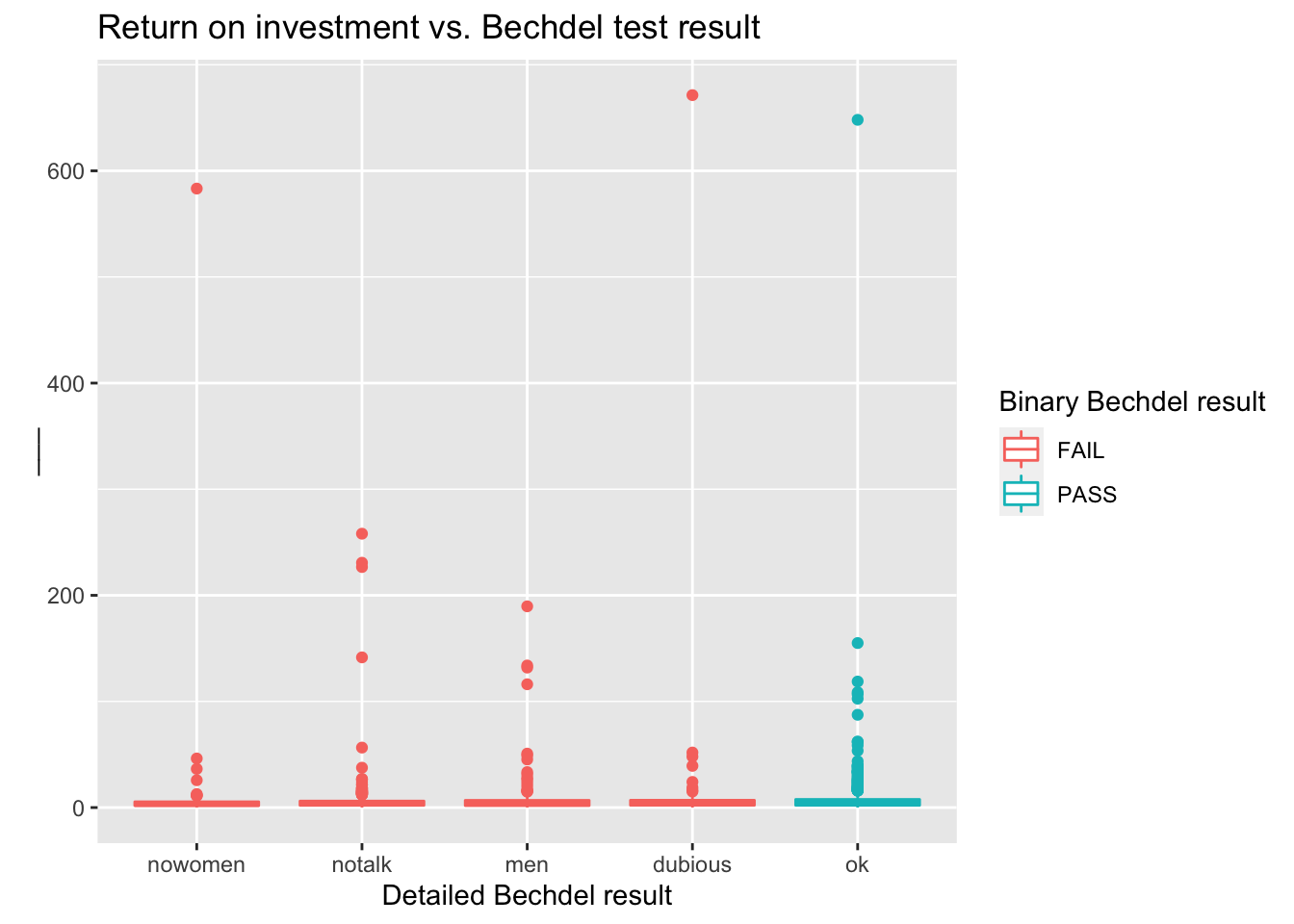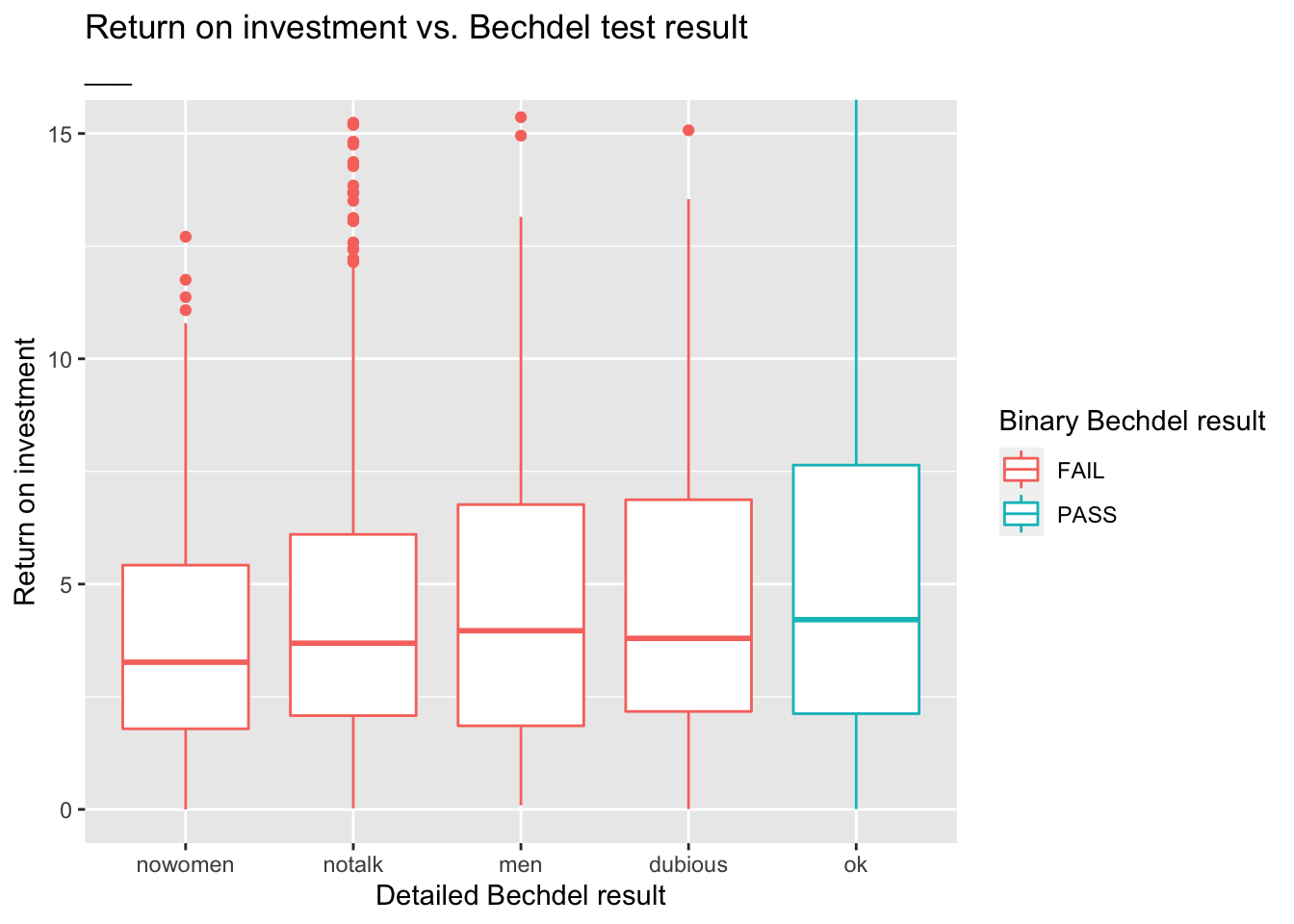# Bechdel

Author

Mine Çetinkaya-Rundel

In this mini analysis we work with the data used in the FiveThirtyEight story titled “The Dollar-And-Cents Case Against Hollywood’s Exclusion of Women”. Your task is to fill in the blanks denoted by `___`.

## Data and packages

``````library(fivethirtyeight)
library(tidyverse)``````

The dataset contains information on 1794 movies released between 1970 and 2013. However we’ll focus our analysis on movies released between 1990 and 2013.

``````bechdel90_13 <- bechdel %>%
filter(between(year, 1990, 2013))``````

There are `___` such movies.

The financial variables we’ll focus on are the following:

• `budget_2013`: Budget in 2013 inflation adjusted dollars
• `domgross_2013`: Domestic gross (US) in 2013 inflation adjusted dollars
• `intgross_2013`: Total International (i.e., worldwide) gross in 2013 inflation adjusted dollars

And we’ll also use the `binary` and `clean_test` variables for grouping.

## Analysis

Let’s take a look at how median budget and gross vary by whether the movie passed the Bechdel test, which is stored in the `binary` variable.

``````bechdel90_13 %>%
group_by(binary) %>%
summarise(
med_budget = median(budget_2013),
med_domgross = median(domgross_2013, na.rm = TRUE),
med_intgross = median(intgross_2013, na.rm = TRUE)
)``````
``````# A tibble: 2 × 4
binary med_budget med_domgross med_intgross
<chr>       <dbl>        <dbl>        <dbl>
1 FAIL    48385984.    57318606.    104475669
2 PASS    31070724     45330446.     80124349``````

Next, let’s take a look at how median budget and gross vary by a more detailed indicator of the Bechdel test result. This information is stored in the `clean_test` variable, which takes on the following values:

• `ok` = passes test
• `dubious`
• `men` = women only talk about men
• `notalk` = women don’t talk to each other
• `nowomen` = fewer than two women
``````bechdel90_13 %>%
#group_by(___) %>%
summarise(
med_budget = median(budget_2013),
med_domgross = median(domgross_2013, na.rm = TRUE),
med_intgross = median(intgross_2013, na.rm = TRUE)
)``````
``````# A tibble: 1 × 3
med_budget med_domgross med_intgross
<int>        <dbl>        <dbl>
1   37878971     52270207     93523336``````

In order to evaluate how return on investment varies among movies that pass and fail the Bechdel test, we’ll first create a new variable called `roi` as the ratio of the gross to budget.

``````bechdel90_13 <- bechdel90_13 %>%
mutate(roi = (intgross_2013 + domgross_2013) / budget_2013)``````

Let’s see which movies have the highest return on investment.

``````bechdel90_13 %>%
arrange(desc(roi)) %>%
select(title, roi, year)``````
``````# A tibble: 1,615 × 3
title                     roi  year
<chr>                   <dbl> <int>
1 Paranormal Activity      671.  2007
2 The Blair Witch Project  648.  1999
3 El Mariachi              583.  1992
4 Clerks.                  258.  1994
5 In the Company of Men    231.  1997
6 Napoleon Dynamite        227.  2004
7 Once                     190.  2006
8 The Devil Inside         155.  2012
9 Primer                   142.  2004
10 Fireproof                134.  2008
# … with 1,605 more rows``````

Below is a visualization of the return on investment by test result, however it’s difficult to see the distributions due to a few extreme observations.

``````ggplot(data = bechdel90_13,
mapping = aes(x = clean_test, y = roi, color = binary)) +
geom_boxplot() +
labs(
title = "Return on investment vs. Bechdel test result",
x = "Detailed Bechdel result",
y = "___",
color = "Binary Bechdel result"
)``````What are those movies with very high returns on investment?

``````bechdel90_13 %>%
filter(roi > 400) %>%
select(title, budget_2013, domgross_2013, year)``````
``````# A tibble: 3 × 4
title                   budget_2013 domgross_2013  year
<chr>                         <int>         <dbl> <int>
1 Paranormal Activity          505595     121251476  2007
2 The Blair Witch Project      839077     196538593  1999
3 El Mariachi                   11622       3388636  1992``````

Zooming in on the movies with `roi < ___` provides a better view of how the medians across the categories compare:

``````ggplot(data = bechdel90_13, mapping = aes(x = clean_test, y = roi, color = binary)) +
geom_boxplot() +
labs(
title = "Return on investment vs. Bechdel test result",
subtitle = "___", # Something about zooming in to a certain level
x = "Detailed Bechdel result",
y = "Return on investment",
color = "Binary Bechdel result"
) +
coord_cartesian(ylim = c(0, 15))``````